# Joachim Despland-Lichtert

### Pathfinding for the Mammoth Project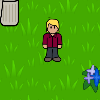While I was working on Mammoth with the McGill Games Research Lab, my project was to develop a new threaded and real-time pathfinding solution for the MMOG that would approach the problem hierarchically and feature caching of common pathways.

The following is an article I originally wrote for the mammoth wiki, describing the work I did:

#### A* Pathfinding

The map of a video game world can be represented using a graph of connected nodes. In older 2D games, the game world is usually represented by an arrangement of tiles (usually squares) on a regular grid. Movement from one tile to another could be achieved depending on whether the tile was an obstacle or not, and this also determined the structure of the graph used for pathfinding (a connection between two nodes exists only if movement is possible between the two corresponding tiles. Note that the problem of pathfinding is not restricted to games in any way, similarly tiled representations of the world are often used in robotics research, and road networks or connections between airports can also represented by graphs on which pathfinding is performed.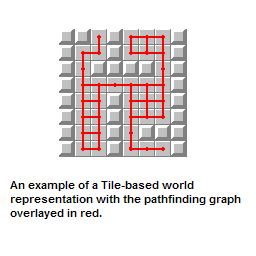The usual most efficient way to find a path on a graph is to use Dijkstra's algorithm, which checks each vertex one by one in order of their distance from the starting vertex. The problem with Dijkstra's algorithm is that it is made with completely arbitrary arrangements of vertices and edges in mind, and assumes that no information is known about the structure of the graph. This, however, is often not the case in real applications, since each node in the graph might represent a point in a known space. For example, if we are using a 2D grid of tiles, we know that each node will have four connections, and the position that would be reached by following them. we can also make the assumption that the shortest path is more likely to be going in the direction of the goal than the opposite.

This idea is the reason why A* is a much better choice than Dijkstra for doing pathfinding in most cases. We use an heuristic function that embodies the information we have about the structure of the graph to decide what node to search next. As an example: if the nodes of the graph represent points in 2-dimensional space, the heuristic for a node would be given by the euclidean distance from it to the goal node.

Another example in a case where we have even more information about the map: If we know that we are looking for a path between two points in the streets of Manhattan, we could use Euclidean distance since we know that the map is a 2D space. However, we also know that all the streets in Manhattan are aligned to two orthogonal axes, so a better Heuristic would be to use Manhattan distance (d = dx+dy) instead, since no diagonal paths are possible.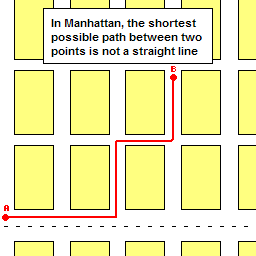Note that when choosing the node to search next in order to find an optimal path, A* should not greedily chose the node that is the closest to the goal but rather consider a function of the heuristic representing the distance of the goal, and the cost to actually get to that node from the start. This function can be written f(n) = h(n) + g(n) where g and h are the heuristic and distance from the starting point. An absolutely excellent explanation of the A* algorithm is available at Amit's website: Note that if the heuristic function is guaranteed to be lower or equal to the minimum possible path from the node to the destination(as is the case if it is the distance between the two since obstacles would only lengthen the path), then A* is guaranteed to find the shortest path. If finding a good enough path faster is more important, then the heuristic should be given more weight than the distance from the starting point.

#### A* in non-tiled space

As we have seen, A* is a very good way of doing Pathfinding in a graph representation of a game world, but what if we have no such representation? In the case where the game world is defined in term of square tiles then it is easy to get such a graph since it is inherent to the way the world is modeled, but in Mammoth, obstacles can be placed at any continuous coordinates on the map and can have any shape. One would think that simply overlaying a grid on the world and removing the nodes that collide with obstacles would be a good idea, but the following five example should show that such an approach causes many problems.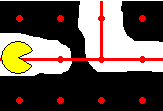Example 1: In a case where there is a very narrow obstacle between two nodes of the graph, none of the two nodes might be in collision, and thus they would stay in the graph and the pathfinder might find a path going from one to the other. Such a path, however, would not be valid since it passes through an obstacle in the world.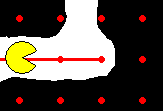Example 2: Another example is that a narrow passage that is barely wide enough to allow an object to pass through would have to be aligned with the grid perfectly in order to be seen by the pathfinding algorithm as a usable passage.

The easiest way to deal with both those problem is to choose a finer grid, which would make them less likely even if it does not eliminate them completely, but doing so would increase the size of the pathfinding task by a large amount, which for real-time applications is highly undesirable. Since Mammoth does not use tiles in its representation of the game world and is therefore subject to those problems, pathfinding performance used to be a large issue.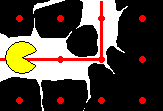Example 3: Assume that a similar passage does align to the grid, but is now too small to let the object doing the pathfinding pass through. This passage would still register as being valid by the pathfinder.

A way to get rid of the problems in Example 3 and Example 1 would be to use the grid to define a tiling of the world space, and instead of eliminating nodes that are in collision with an obstacle, eliminate the node whose surrounding tile contains any obstacle. Doing this, however, would amplify the problem of Example 2 hugely, unless the grid was again much finer.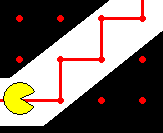Example 4: Another problem is that when we represent a continuous 2D map with a grid, the paths must be composed of individual edges of the graph. If our graph is a square grid and the optimal path is a diagonal, the path returned will be a zig zag from the starting point to the destination, which is very unefficient. A fix would be to add diagonal edges between nodes, but this still limits the paths to moving in eight directions and would increase the search space as well.

Example 5: Finally, in a large world such as the one modeled in a MMO, generating and storing the graph might be extremely expensive, especially since the obstacles are not aligned to tiles, which as we have seen means that the grid must be very fine. In addition, if we assume that obstacles can change as the game is running, then rebuilding the grid might be necessary. Finally, different objects might require separate graphs for pathfinding since a passage might be wide enough for one but not the other.

#### A solution to all these problems

Mammoth does not generate or store any pathfinding graph: When a pathfinding is called, only the start and destination positions are known. Instead of searching a pregenerated map, a graph is built on the fly while doing the search by taking a small step in the 4 directions around each node that is searched and creating new nodes where no collisions are found. In this way, only the part of the graph that the algorithm needs to search to find a path has to be stored in memory. This also allows pathfinding to be done on any number of different “grid” types without additional cost, since it does not rely on a graph that was generated at startup. It also means that it is not necessary to regenerate a grid when obstacles change (for example a door becomes locked).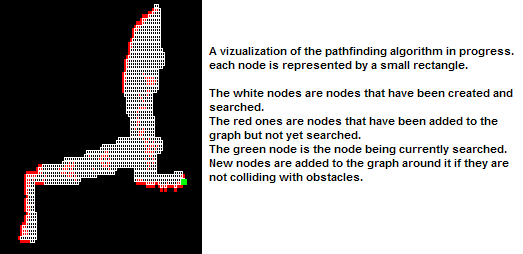Another way that the above feature is exploited is that when any object calls a pathfinding, the graph that is generated on the fly will align to a grid that depends on the size of the object. If a very small object calls a pathfinding, the grid used to generate the nodes needed will be very fine, and thus allow the object to find paths through small openings. If the object is very large on the other hand, the grid does not need to be as small so performance is always optimal.

Note that this feature could also be used in a case where different objects have different pathfinding abilities: a character that can swim would need a different graph than one that cannot for example, and this prevents any additional costs in such cases.

The graph will also use the shape of the object itself to do the collision tests at each node in the graph, in order to make sure that there are no problems where large objects think they can find a path through a narrow opening because the grid was aligned with it.

Another feature is the use of swept areas for collision detection: Instead of simply testing if the shape of an object intersects with an obstacle at every step, a new shape is calculated which corresponds to the area swept by the object between its current position and the one at the last step. This new shape is then the one used for testing the collision. This prevents most of the issues mentioned in the examples since the swept area will collide with any obstacle present in the path of the object between the two steps.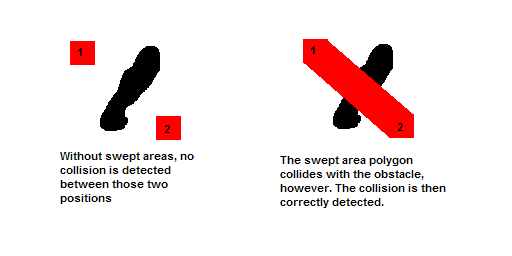Swept areas are also used to enable another nice feature: Path smoothing. After finding a valid path, it is optimized and any unnecessary zig zags are eliminated. This is possible because we can use swept areas to test for collisions between two waypoints in the path, and simply eliminate the unnecessary intermediate waypoints if no collision is found.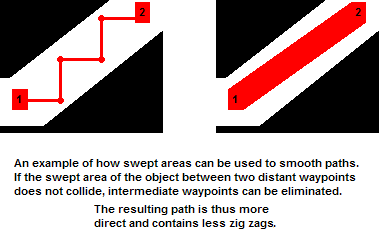An other important feature of the pathfinding in Mammoth is that it is done in a separate thread while the game is running. This in itself is not a huge improvement since an object would still have to wait until a path is found before it can start following it. If the path is very complex and the destination far away, this could take quite some time. The way this is handled is by allowing the pathfinding algorithm to do a search for only a short amount of time (less than a second), after which, if no path has been found, the object will start following the path to the best waypoint reached, which is very likely to be in the correct direction. While the object is moving along this path approximation, a new pathfinding is running in the background, and attempts to find the correct path to the destination from that last waypoint. If the object reaches the waypoint before the final path has been found and sufficient progress has been made in the search, then the process repeats itself.

#### Caching of common path segments

As the game keeps running and different objects use pathfinding to move around the map, a lot of searches might be redundant since they search through the same waypoints over and over again. A solution to this is to save the path segments that have been recently used in a data structure so that they can be used later. When path smoothing is done, the segments that result are cached into that structure so that they can be used on subsequent searches as “shorctuts” (skipping the intermediate steps of the search that resulted in this segment). The segments that are kept in the cache correspond to those that are used the most often, but also those that cover the longest distance, since they would be more useful in terms of shortening the searches. When doing the search, exploring these shortcuts is preferred (by an adjustable factor) over taking regular steps, since they are expected to be more useful and are known to be valid.

#### Hierarchical Pathfinding

The best way to make the pathfinding faster and more efficient is to find a better Heuristic function. If it was possible to somehow find a heuristic that would take into account the obstacles in the way to the goal, pathfinding would be much more efficient since the search would not get stuck into dead ends simply because they are going in the direction of the goal (which is what happens if distance is the heuristic used).

If you think about the way you do pathfinding when you want to get somewhere, you usually do not plan out every step of the way to the goal and consider every small obstacle you might encounter along the way. You probably start by thinking about higher-level concepts such as roads or streets and find a path using those first, then start walking in the direction of that high-level path, considering low-level obstacles that stand in your way as you go along.

A similar approach can be used to make pathfinding more efficient. A high-level graph representation of the world can be generated by using a relatively sparse tiling, and connecting the nodes corresponding to the tiles if a direct path exists from one tile to the other. Note that in this case we are not worried about small obstacles standing in the way. The straight line from one node to the other might encounter a collision, but we still connect the nodes as long as a path exists. If we do pathfinding on this graph, it results in a high-level path that considers large-scale features of the map, but ignores the small obstacles that the object would have to find a low-level path around.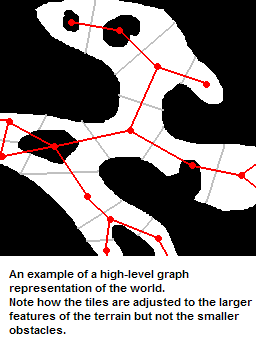This high-level path can then be used as a heuristic for our main (low-level) pathfinding algorithm, for example by using the distance remaining in the high-level path instead of the straight-line distance. This can greatly improve performance since it is a much better estimate.

Finally, it is entirely possible to have an even-higher level representation of the world in order to have a better heuristic to find a path in the high-level graph. Depending on the size of the world, there is no limit to the number of hierarchical levels of abstraction you can use to improve pathfinding performance.